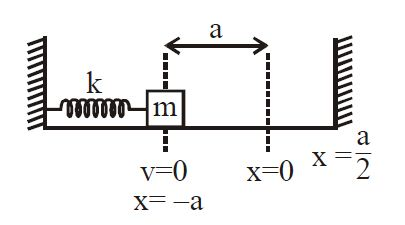# Collision in Spring Mass System!A spring (spring constant $k$) having one end attached to rigid wall & other end attached to a block of mass $m$ kept on a smooth surface as shown in figure. Initially spring is in its natural length at $x = 0$, now spring is compressed to $x = -a$ and released. (Coefficient of restitution $e = \dfrac 1 2$ ). If velocity of block just after first collision is $a\sqrt{\frac{nk}{16m}}$ . Find the value of $n$.

×